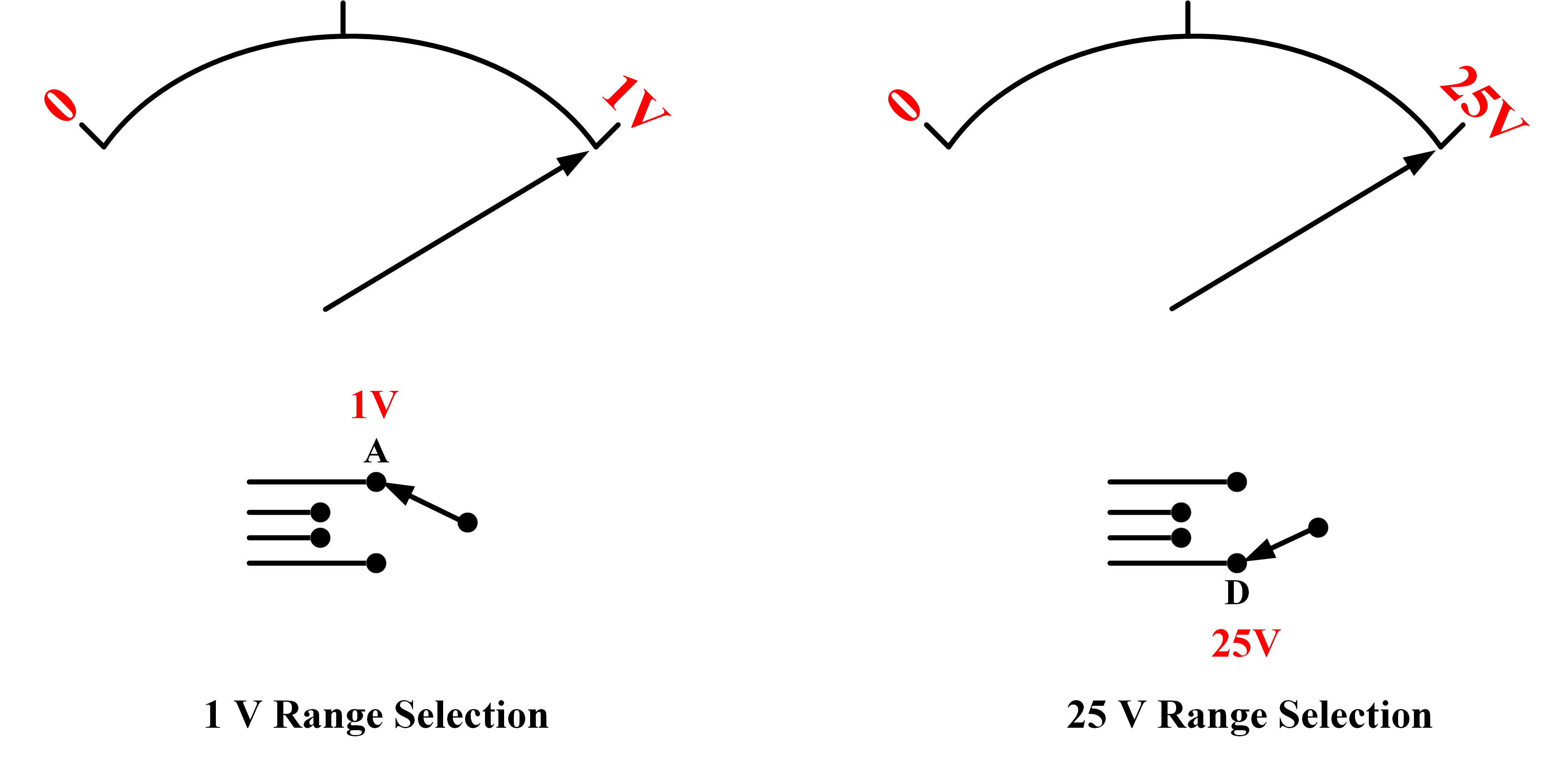# Electronic Voltmeter Working and Block Diagram

Want create site? Find Free WordPress Themes and plugins.

An analog electronic voltmeter uses an electronic amplifier to improve the performance of an electromechanical voltmeter. For example, an electronic voltmeter has a much higher input resistance than an electromechanical instrument, so voltmeter loading effect is considerably reduced.

Also, voltage levels that are normally too small for measuring on an electromechanical instrument can be amplified to measurable levels in an electronic instrument.

## Electronic Voltmeter Working

The basic circuit of one type of analog electronic voltmeter is illustrated in figure 1. This particular circuit is made up of three stages: an input attenuator, an electronic amplifier, and an electromechanical voltmeter stage.Fig.1: Electronic Voltmeter Circuit Diagram (Block Diagram)

Note the large triangular graphic symbol normally used to represent an amplifier. Also, note the small triangular symbol representing the instrument ground.

The input attenuator is simply a voltage divider that divides (or attenuates) high input voltages to measurable levels.

The ampliﬁer has a very high input resistance so that there is virtually no loading effect on the attenuator resistors. It also has a low output resistance to supply the current required by the electromechanical voltmeter stage.

Amplifier

The ampliﬁer has voltage gain (or amplification) of 1, which means that a 1 V input produces a 1 V output. So, its function is solely to offer a high input resistance and a low output resistance. In this situation it is said to be a buffer between the attenuator and voltage-measuring stages; thus, it is termed a buffer ampliﬁer.

A DC supply voltage (Vcc) must be provided for the ampliﬁer, and this may be derived from a battery or power supply contained within the instrument. The operation of the ampliﬁer cannot be understood until electronic devices are studied.

Electromechanical Voltmeter Stage

The electromechanical voltage-measuring stage is typically designed to give meter FSD for an ampliﬁer output of 1 V. Because the ampliﬁer has a gain of 1, its output voltage (V0) is equal to the input (Vi) from the attenuator. Thus, meter FSD is obtained when the attenuator produces a 1 V output.

Attenuator

The attenuator switch is the voltmeter range-selection switch. With the switch at position A, an attenuator input of 1V is passed to the voltage measuring-stage to give FSD. This (1V) is the maximum input voltage that can be measured when the switch is at terminal A. Thus, position A of the range-selection switch is identified at the 1V range position [see Figure 2].Fig.2: Electronic Voltmeter Range Selection

When the selection switch is at position D, the voltage-divider theorem gives the attenuator output as:

${{V}_{i}}=E\times \frac{{{R}_{4}}}{{{R}_{1}}+{{R}_{2}}+{{R}_{3}}+{{R}_{4}}}$

${{E}_{\max }}={{V}_{i}}\times \frac{{{R}_{1}}+{{R}_{2}}+{{R}_{3}}+{{R}_{4}}}{{{R}_{4}}}$

For FSD, Vi =1V. Therefore, the maximum input voltage is

${{E}_{\max }}=1\times \frac{800+100+60+40}{40}=25V$

As illustrated in figure 13-11 (c), position D of the switch gives a voltmeter range of 25V.

## Electronic Voltmeter Example

Calculate the input resistance of the voltmeter in figure 13-11 (a) if the amplifier input resistance has no effect on the attenuator. Also, determine the voltmeter range at positions B and C of the range-selection switch.

Solution

\begin{align} & R={{R}_{1}}+{{R}_{2}}+{{R}_{3}}+{{R}_{4}} \\ & =800+100+60+40=1\text{M }\!\!\Omega\!\!\text{ } \\\end{align}

At position B,

${{E}_{\max }}={{V}_{i}}\times \frac{{{R}_{1}}+{{R}_{2}}+{{R}_{3}}+{{R}_{4}}}{{{R}_{2}}+{{R}_{3}}+{{R}_{4}}}$

${{E}_{\max }}=1\times \frac{800+100+60+40}{100+60+40}=5V$

At position C,

${{E}_{\max }}={{V}_{i}}\times \frac{{{R}_{1}}+{{R}_{2}}+{{R}_{3}}+{{R}_{4}}}{{{R}_{3}}+{{R}_{4}}}$

${{E}_{\max }}=1\times \frac{800+100+60+40}{60+40}=10V$

The solve advantage of the type of electronic voltmeter discussed above (compared to an electromechanical voltmeter) is that it has a high input resistance (1MΩ). However, the instrument range can be extended to measure low-voltage levels by arranging for the amplifier to have a voltage gain greater than 1. For example, if the amplifier has a precise gain of 10, an input of 100 mV to the attenuator produces an amplifier output of 1V. So, the instrument scale can be calibrated for an FSD of 100 mV.

Did you find apk for android? You can find new Free Android Games and apps.